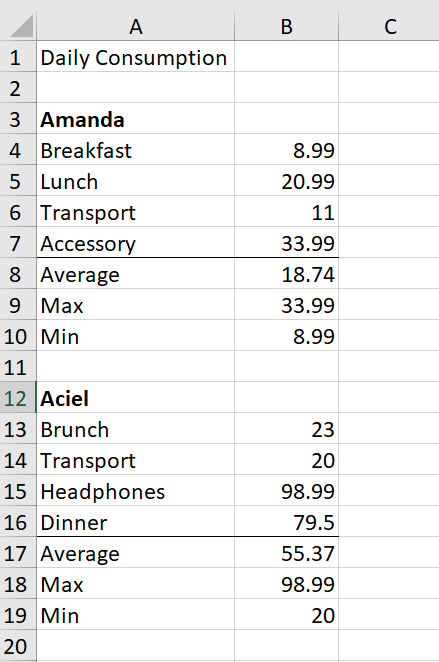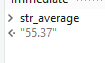# Extract a certain figure in excel without stating the cell position

I want to extract the average spending of Aciel only, is it possible to do it without stating the exact cell position? Thanks.Hi @Katrina

To get the row Index

``````"A"+(index+2).ToString
``````

Try this below expression to get the AVG

``````DtData.AsEnumerable(). Average(Function(row) Cint(row (1)))
``````
``````(From d in dtData.AsEnumerable Where Not (isNothing(d(1)) OrElse String.IsNullorEmpty(d(1).toString.Trim))Select v = CDbl(d(1).toString.Trim)).Average(Function (x) x)
``````

Regards
Gokul

1 Like

Hi

1. Use a read range activity and get the output as DATATABLE named dt

2. Then use a assign activity like this

stroutput = dt.Asenumerable().Where(Function(a) a.Field(of String)(“Daily Consumption”).ToString.Contains(“Average”)).CopyToDatatable().Rows(0)(1).ToString

For more details on DATATABLE and it’s expression

Cheers @Katrina

Hi @Katrina ,

Here is an approach which works for me.
First find the index of the Aciel using this:

``````Dt.AsEnumerable().Where(Function(w) w.ItemArray.Contains("Aciel")).Select(Function(s) Dt.Rows.IndexOf(s)).First()
``````

Once you have the index, now you can read the range once more with range as

``````"A"+(index+2).ToString
``````

Similar approach for finding the Average:

``````Dt.AsEnumerable().Where(Function(w) w.ItemArray.Contains("Average")).Select(Function(s) Dt.Rows(Dt.Rows.IndexOf(s))(1).ToString).First()
``````

If you could provide a sample dataset, I can provide you with a sequence that encapsulates the entire logic.

Kind Regards,
Ashwin A.K

1 Like

Daily Consumption.xlsx (9.9 KB)

Hi @Katrina ,

Here are the results:To retrieve index of Aciel →

``````dt_sampleData.AsEnumerable().Where(Function(w) w.ItemArray.Contains("Aciel")).Select(Function(s) dt_sampleData.Rows.IndexOf(s)).First()
``````

The Range is read from Aciel onwards and overwritten into our DataTable variable.
Next, we retrieve the Average from Aciel →

``````dt_sampleData.AsEnumerable().Where(Function(w) w.ItemArray.Contains("Average")).Select(Function(s) dt_sampleData.Rows(dt_sampleData.Rows.IndexOf(s))(1).ToString).First()
``````

GetDataBasedOnKeyword.xaml (6.5 KB)

Kind Regards,
Ashwin A.K

Solved, thx a lot.

1 Like

This topic was automatically closed 3 days after the last reply. New replies are no longer allowed.